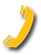## The Pile Integrity Test (PIT) method can be utilized to determine the unknown length of a concrete deep foundation. The pile length is calculated from the time between the impact and the first sharp positive velocity reflection (assuming a reasonable wave speed of around 12,500 ft/s). The formula is as follows:

Length = (Time x 12,500 ft/s) / 2

The stress wave speed value is used to convert the measured stresswave travel time to distance from the accelerometer location near the pile top. A reasonable value of 12,500 ft/s is assumed for concrete foundations. Since the actual stresswave speed may vary by as much as +/-5%, depending on various factors, a similar variation in calculated distances should be recognized.

## The Parallel Seismic Method is another method that could be utilized to determine the unknown length of a deep foundation. This method is performed by installing a borehole adjacent to the deep foundation in question. The borehole must be parallel to the foundation element and within a few feet proximity. The borehole should also extend about 10-15 feet below the expected bottom of the foundation.

The top of the foundation is impacted with an impulsive hammer that generates compressional and/or shear waves that travel down the foundation and into the surrounding soil. The waves are collected at various intervals along the borehole using a hydrophone receiver probe and plotted versus depth.

The foundation length is determined from the first arrival time of the signal. In general, the signal will travel faster through the foundation element then the soil. Therefore, when the hydrophone is below the bottom of the foundation, the signal first arrival time will be incrementally delayed versus depth at a higher rate than when the hydrophone is adjacent to the foundation. The location of the change in slope is said to be the bottom of the foundation.

## Parallel Seismic Testing (PST Test):